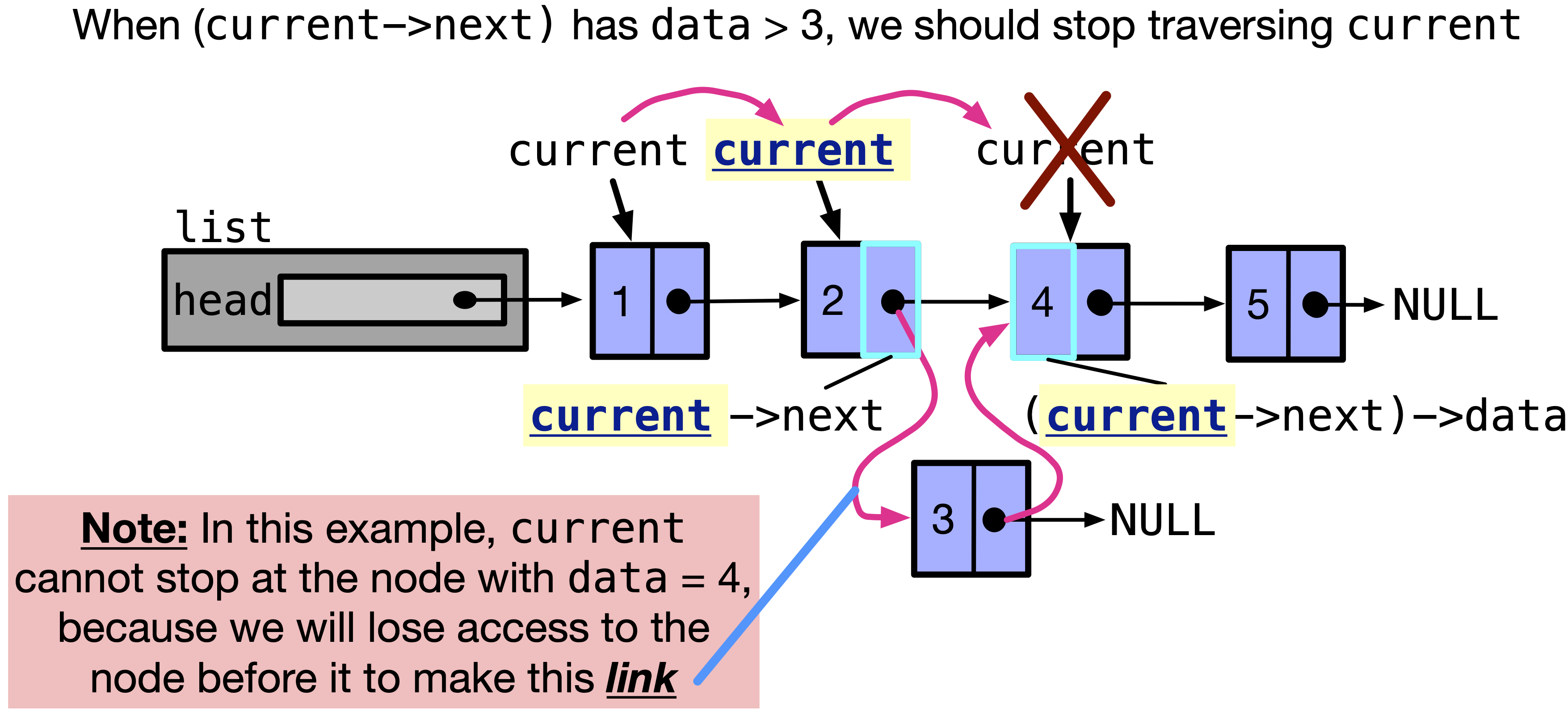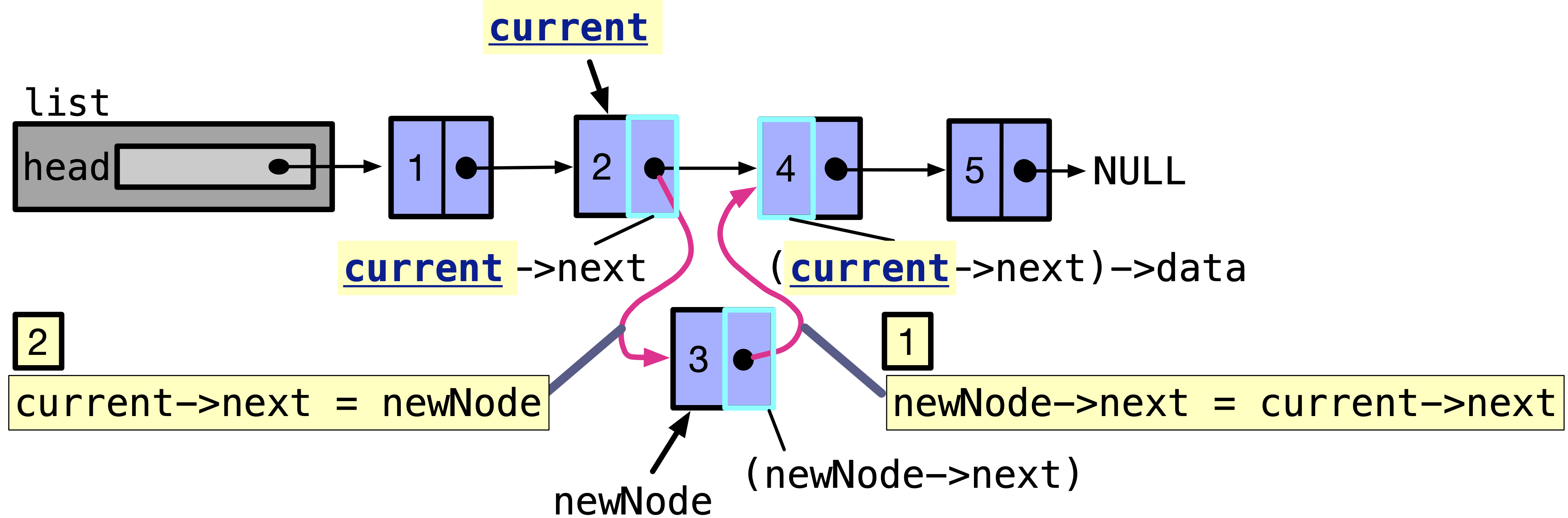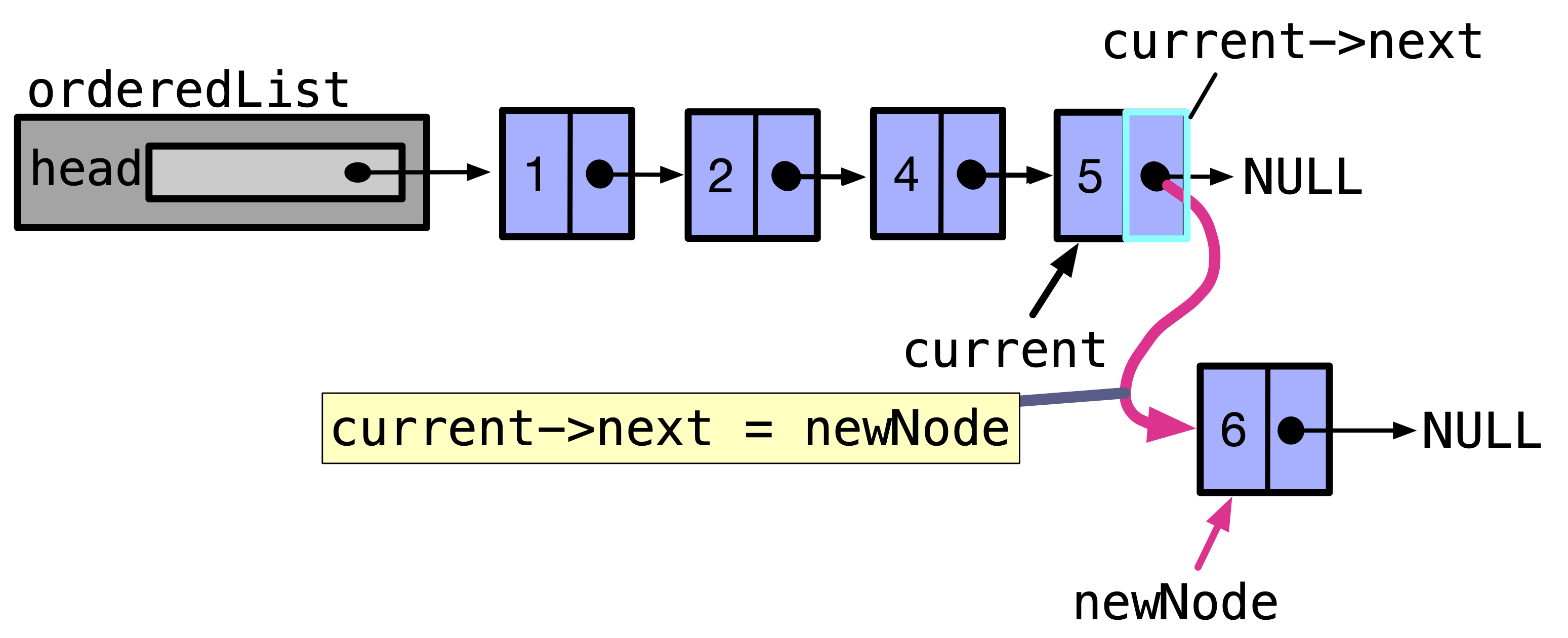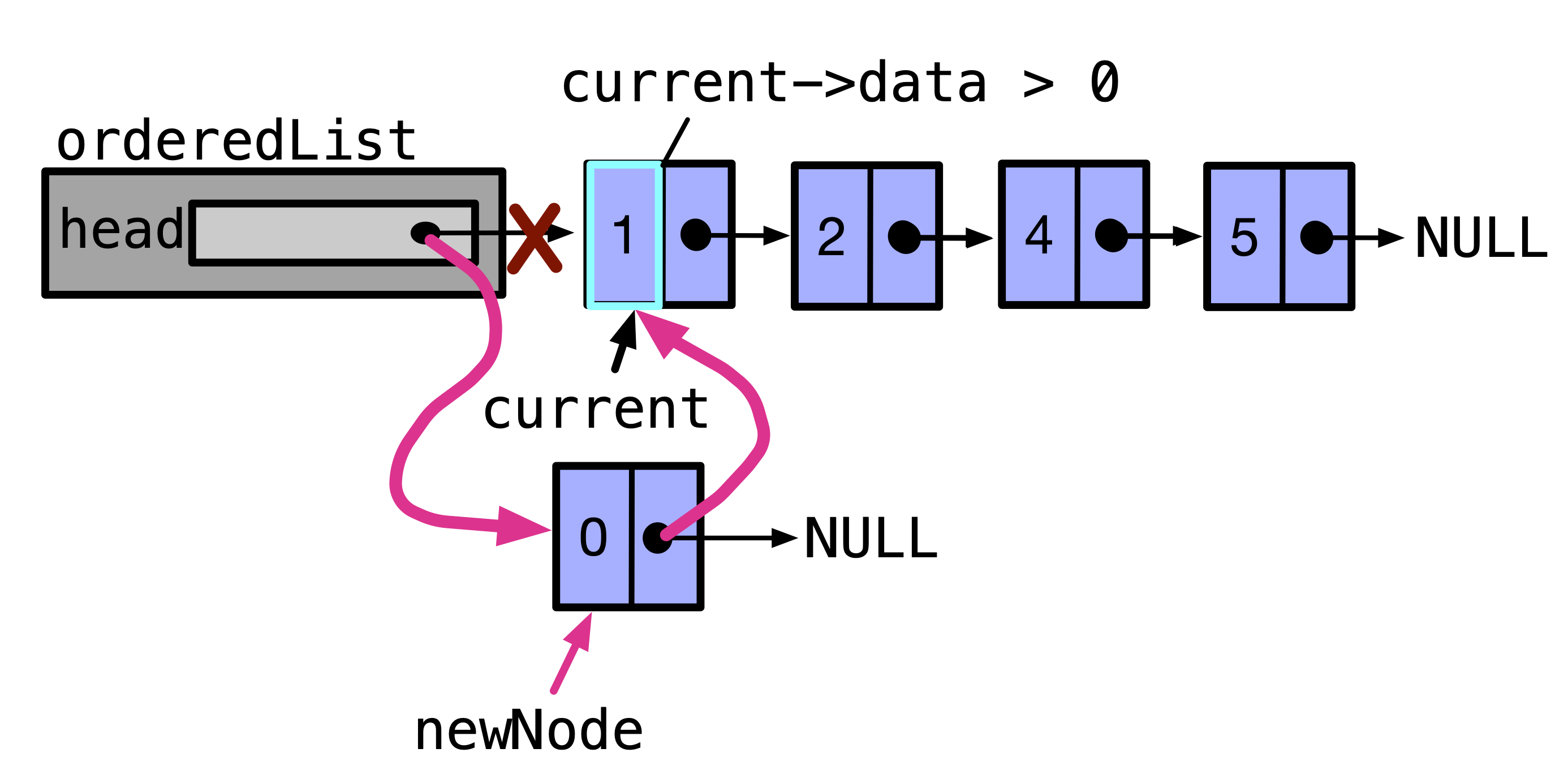# 13.3. Insert nodes into a linked list#

In this section, we will discuss how to insert nodes into a linked list. We will discuss how to insert a node at the beginning of the list, at the end of the list, and in the middle of the list.

## 13.3.1. Preliminaries#

Before we implement any functions, let’s recap the data structure we will be using. We will be using the following Node structure:

typedef struct node {
int data;
struct node *next;
} Node;


## 13.3.2. Create a node in the linked list#

In the previous section, we discussed how to create a linked list. Before inserting any node, we had to dynamically allocate it first. We can do so by using the malloc function. The malloc function takes in the size of the memory space we want to allocate, and returns a pointer to the memory space. We can then assign the pointer to a pointer variable of type Node.

malloc returning NULL

If malloc returns NULL, it means that it was unable to allocate memory. This can happen if the program is running out of memory. Hence, we should check if the pointer returned from malloc is NULL before we use it. If it is NULL, we should return NULL from the function.

If we use it anyway, we will get a segmentation fault. Segmentation fault is a common error that occurs when we try to access memory that we are not allowed to access. This can happen if we try to access memory that is not allocated to us, or if we try to access memory that has been freed. For example, if newNode is NULL, and we do newNode->data = 1, it will cause segmentation fault, since newNode is not pointing to any memory space.

We can use createNode to form the following linked list:

Download the following code createNode-example.c if you want to play with it.

Code

Output

1 -> 2 -> 4 -> 7.


In line $$14$$, we call createNode which returns a pointer to a newly dynamically allocated Node with data set to 1. We then assign the address of this Node to head.

In line $$16$$, we call createNode again, which returns a pointer to a newly dynamically allocated Node with data set to 2. The mistake is to set the address of the newly allocated Node to head. The problem is that no other pointer will have the address of the Node with data set to 1. This is a memory leak, since we can never access that previously allocated node.

Instead, in line $$17$$, we can call createNode again, which returns a pointer to a newly dynamically allocated Node with data set to 2. We then set the next pointer of the head to point to this newly allocated Node, e.g. head->next = createNode(2);. This way, we link the two nodes together, where head is pointing to the first node, and head->next is pointing to the second node.

In line $$19$$, we call createNode again, which returns a pointer to a newly dynamically allocated Node with data set to 4. We then set the next pointer of the second node head->next to point to this newly allocated Node, e.g. head->next->next = createNode(4);. This way, we link the three nodes together, where head is pointing to the first node, head->next is pointing to the second node, and head->next->next is pointing to the third node.

In line $$20$$, we call createNode again, and set its return value to head->next->next->next to link the four nodes together.

Note: Of course the above code is missing the free statements to free the memory allocated to the nodes. We will discuss this in the next section.

Too many ->next

It can be confusing to keep track of ->next numbers in a statement. Instead, we use different functions to insert nodes at the beginning, end, and middle of the list.

## 13.3.3. Inserting a node at the beginning/front of the list#

To insert a node at the beginning of the list, we need to create a new node, and set its next pointer to point to the current head. We then set the head to point to the newly created node. The steps are illustrated in the following figure.

We can implement a function to insert a node at the front of the list. The function can take the head of the list and the data of the node to be inserted as parameters. The function will return a bool that is true if the node was successfully inserted, false otherwise. The following figure illustrates the function and draws the changes to the linked list at each step.

Errorneous Code

Issues with passing head by value

In the above code, we pass head by value, and head becomes a local variable holding the address of the first node of the list in insertAtFront function. Even if we change the value of head in insertAtFront, it will only change in insertAtFront function. It will not change the value of head in main function. Hence, the linked list will not be changed.

The following figure shows how head gets changed in insertAtFront function, but not the main function.

### 13.3.3.1. Solution 1: Pass pointer to head#

To fix this issue, we can pass a pointer to head to insertAtFront function. The following figure shows how we can pass head as a pointer.

 1bool insertAtFront(Node **headPtr, int value) {
2  Node *temp = createNode(value);
3  if (temp == NULL) {
4    return false;
5  }
8  return true;
9}
10
11int main(void) {
14
16
17  return 0;
18}


In line $$1$$, we receive a pointer to Node* head variable. This is a double pointer, hence the type is Node**. We can use this pointer to change the value of head in main function.

In line $$6$$, we set temp->next to point to the current head. The current head is pointed to by Node** headPtr. We can dereference headPtr to get to the current head in the main function. Hence, we set temp->next =  *headPtr;.

In line $$7$$, we change the head in main function to point to the newly created node pointed to by temp. We can dereference headPtr to get to the current head in the main function. Hence, we set *headPtr = temp;.

In line $$15$$, we call insertAtFront function to insert a node with data set to 0 at the beginning of the list. We pass the address of head to insertAtFront function as &head. Hence, headPtr in insertAtFront function will point to head in main function. We can then change the value of head in main function.

The following figure illustrated the value of variables in main function before and after calling insertAtFront function.

### 13.3.3.2. Solution 2: Create a new data structure#

Since double pointers can be confusing to deal with, we can create a new data structure to hold the head of the list. Then, pass a pointer to the data structure if we want to change the head.

Let’s first discuss how does the new data structure look like. It looks as follows:

typedef struct list {


We can then create a new data structure in main function. The following figure shows how we can create a new data structure in main function.

Code

We can then pass a pointer to the data structure LinkedList to the insertAtFront function. This serves as a pointer to the Node* head pointer holding the address of the first node in the list. The following figure shows how we can pass a pointer to the data structure LinkedList to the insertAtFront function.

Code snippet

## 13.3.5. Insert a node at the end of the linked list#

If we want to insert a node at the end of the linked list, we have to traverse till the last node, and insert the new node at the next of the last node. The following figure shows how we can insert a node at the end of the linked list.

To implement the function that inserts a node with data = value at the end of the linked list, we can take LinkedList *list and int value as input, and return bool, which is true if the node was inserted successfully, and false otherwise. In the function, we will traverse the linked list with a Node* pointer named current and stop when current->next is equal to NULL. This is when current reached the end of the list. Then, we can insert the new node at the next of current. The following figure shows how we can implement the function that inserts a node at the end of the linked list.

Missing a case: Code snippet

However, the figure above is missing one case. What if the linked list is empty? current will be NULL, and we will get segmentation fault if we do current->next. Hence, before checking the next of current, if current was NULL, we just make list->head point to that new node. The following code shows how we can handle the case when the linked list is empty.

Code

 1bool insertAtBack(LinkedList *list, int value) {
2  Node *temp = createNode(value);
3  if (temp == NULL) {
4    return false;
5  }
7
8  if (current == NULL) {
9    // The list is empty, there is no difference between back/front.
11    return true;
12  }
13
14  // Traverse the list until we reach the last element.
15  while (current->next != NULL) {
16    current = current->next;
17  }
18
19  // current now points to the last element of the list.
20  current->next = temp;  // Add a new node to the end.
21  return true;
22}


In line $$8$$ to $$12$$, we handle the case if the list was empty. If the list was empty, we just make list->head point to the new node. u

## 13.3.6. Insert a node into an ordered linked list#

To implement the function that inserts a node with data = value into an ordered linked list, we can take LinkedList *list and int value as input, and return bool, which is true if the node with data equal value was inserted successfully, and false otherwise.

We are given a list sorted in ascending order according to the value of data. If we want to insert a node into an ordered linked list, we have to traverse the linked list till we find the node that has data greater than the data of the new node. Then, we can insert the new node before that node.

In the function, we will traverse the linked list with a Node* pointer named current. We should stop at the node before inserting the new node. This is when current->next->data is greater than value. Then, we can insert the new node between current and current->next. We cannot stop current at the node with data > value, because we will lose access to the previous node after which we should insert our node. The following figure shows how we can traverse the linked list with the pointer current to stop at the node after which our new node will be inserted.Fig. 13.2 When traversing the linked list, we need to stop just before where we want to insert our node. The following figure shows where should we stop, when we insert a node between two nodes.#

When current is pointing to the node after which we will insert the new node, we can now (i) link the next of new node to the next of current: newNode->next = current -> next, and (ii) link the next of current to the newNode: current->next = newNode;. Obviously, we need to first link the next of new node to the next of current, because we do not want to lose access to the node after current.Fig. 13.3 When current is pointing to the node after which we will insert the node, we can now (i) link the next of new node to the next of current: newNode->next = current -> next, and (ii) link the next of current to the newNode: current->next = newNode;.#

The following code shows how we can implement the function that inserts a node between 2 nodes into an ordered linked list.

However, a segmentation fault would happen if we try accessing current->next->data when current is NULL or when current->next is NULL. This can happen in several cases:

1. current will be NULL, when the linked list is empty and we will get segmentation fault if we do current->next.

2. current->next will be NULL, when current is pointing at the last node in the linked list. This happens when current->next->data was always < value, and current is now pointing to the last node. We will get segmentation fault if we do current->next->data.

3. current->next will be NULL, when current is pointing at the first node and only node in the list.

Special case 1: The linked list is empty. Before checking the condition of the while loop, which is current->next->data < value, we need to check if current is NULL. If current is NULL, we just make orderedList->head point to that new node. We need to create the node first and point to it by newNode, then make orderedList->head = newNode. We return true is the node is successfully created, false otherwise. This all can be made by calling insertAtFront or insertAtBack, since the list is empty. The following code shows how we can handle the case when the linked list is empty.

Code

 1bool insertIntoOrderedList(LinkedList *orderedList, int value) {
3
4  if (current == NULL) {
5    // The list is empty, insertion at front/back is the same thing.
6    return insertAtFront(orderedList, value);
7  }
8
9  while (current->next->data < value ) {
10    // The value to insert is larger than the next element in the list.
11    // Move to the next element in the list.
12    current = current->next;
13  }
14
15  Node *newNode = createNode(value);
16  if (newNode == NULL) {
17    // Could not allocate memory for a new node.
18    return false;
19  }
20
21  // current may be the last element in the list, and it may also be the last
22  // element in an ordered list that is less than value.
23
24  // Link the rest of the list with this new node.
25  newNode->next = current->next;
26  current->next = newNode; // Overwrite next with the new node.
27
28  return true;
29}


Special case 2: The value of the new node is greater than the value of the last node in the linked list. This will make the present implementation in lines $$9$$ to $$12$$ loop till current points to the last node in the linked list. After that if we check the condition of the loop current->next->data, we get a segmentation fault since current->next is NULL. We can handle this case by checking if current->next is NULL before checking current->next->data. If current->next is NULL, we just make current->next point to the newNode: current->next = newNode. This is after creating the node first and point to it by newNode. We return true if the node is successfully created, false otherwise. The following figure shows what we can do to insert the node at the tail of the linked list, when the value of the new node is greater than the value of the last node.Fig. 13.4 When current->next is NULL, we just make current->next point to the new node.#

We can do these steps by stopping the while loop when current->next is NULL. The following code shows how we can handle the case when the value of the new node is greater than the value of the last node in the linked list.

Code

 1bool insertIntoOrderedList(LinkedList *orderedList, int value) {
3  if (current == NULL) {
4    // The list is empty, insertion at front/back is the same thing.
5    return insertAtFront(orderedList, value);
6  }
7
8  while (current->next != NULL && value > current->next->data) {
9    // The value to insert is larger than the next element in the list.
10    // Move to the next element in the list.
11    current = current->next;
12  }
13
14  Node *newNode = createNode(value);
15  if (newNode == NULL) {
16    // Could not allocate memory for a new node.
17    return false;
18  }
19
20  // current may be the last element in the list, and it may also be the last
21  // element in an ordered list that is less than value.
22
23  // Link the rest of the list with this new node.
24  newNode->next = current->next;
25  current->next = newNode; // Overwrite next with the new node.
26
27  return true;
28}


In line $$8$$, we check if current->next != NULL first, then only if true, current->next->data < value will be evaluated. Remember this is because in lazy evaluation if the first condition in an && is false, the second condition will not be evaluated. This is because the whole expression will be false anyway. Hence, if current->next == NULL, we will exit the loop without checking current->next->data < value.

Before line $$24$$, newNode->next is set to NULL, and current->next will be NULL if we exited the while loop because current->next == NULL. Hence, in line $$24$$, when we do newNode->next = current->next; we are not doing anything, since newNode->next is already NULL. This line is mainly essential when we insert a node between two nodes, not when we insert at the tail of the linked list.

In line $$25$$, we set current->next = newNode; to link the last node in the linked list to the new node.

current->next can be NULL also if we have only one node in the list. If the data of the new node is larger than the data of the only node, we should insert the new node at the tail of the linked list. Our current implementation makes this happen. However, if the data of the new node is smaller than the data of the only node, we should insert the new node at the front of the linked list. Our current implementation does not handle this case. We will handle this case in special case 3.

Special case 3: The value of the new node is less than the value of the first node in the linked list. If our new node is to be inserted before the first node, because value is smaller than the data of the first node, our code does not cover that.

Before checking if the second node has a value greater than the value we want to insert using current->next->data, we need to check the first node data. If the value of the first node was larger than the value we want to insert, then we need to insert the new node at front. The following figure shows what we need to do to handle this case.Fig. 13.5 When the value of the new node is less than the value of the first node in the linked list, we need to insert the new node at front.#

We can do this by checking if current->data > value before checking current->next->data. If true, we call insertAtFront function. The following code shows how we can handle the case when the value of the new node is less than the value of the first node in the linked list. Download the following code insertIntoOrderedList.c if you want to play with the code.

Code

 1bool insertIntoOrderedList(LinkedList *orderedList, int value) {
3  if (current == NULL) {
4    // The list is empty, insertion at front/back is the same thing.
5    return insertAtFront(orderedList, value);
6  }
7
8  if (current->data > value) {
9    // The value to insert comes before the current head, so insert before it.
10    return insertAtFront(orderedList, value);
11  }
12
13
14  while (current->next != NULL && value > current->next->data) {
15    // The value to insert is larger than the next element in the list.
16    // Move to the next element in the list.
17    current = current->next;
18  }
19
20  Node *newNode = createNode(value);
21  if (newNode == NULL) {
22    // Could not allocate memory for a new node.
23    return false;
24  }
25
26  // current may be the last element in the list, and it may also be the last
27  // element in an ordered list that is less than value.
28
29  // Link the rest of the list with this new node.
30  newNode->next = current->next;
31  current->next = newNode; // Overwrite next with the new node.
32
33  return true;
34}


## 13.3.7. Exercise: Find a node in the linked list#

Let’s practice finding a node with a particular value into an ordered linked list. We need to implement a function that takes the value of the node we want to find, and returns a pointer to the node with that value. If the node is not found, we return NULL. We also need to pass a pointer to LinkedList as a parameter that has a pointer to the head of the linked list.

The following figure shows the function we need to implement to find a node with a particular value in the linked list. Download findFirstNode.c if you want to play with the code.

0 Questions

X